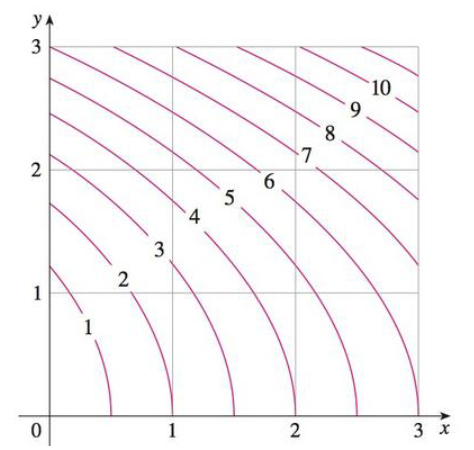Chapter 15, Problem 2RE

Chapter
Section
Textbook Problem

Use the Midpoint Rule to estimate the integral in Exercise 1.1. A contour map is shown for a function f on the square R = [0, 3] × [0, 31. Use a Riemann sum with nine terms to estimate the value of ∬ R f ( x , y )   d A . Take the sample points to be the upper right comers of the squares.To determine

To estimate: The value of given double integral in Exercise 1 by using midpoint rule.

Explanation

Formula used:

The double integral, Rf(x,y)dAi=1mj=1nf(x¯i,y¯j)ΔA ,

Where, ΔA=a2 , where a is the side of each square.

The given function is f(x,y) .

The mid points of each rectangle is denoted by (x¯i,y¯j) , where x¯i is the midpoint of [xi1,xi] and y¯i is the midpoint of [yj1,yj] .

The Riemann sum constants are denoted by m, n.

Given:

The rectangular region, R=[0,3]×[0,3] .

The Riemann sum constants, m=3,n=3 .

Calculation:

Plot the given rectangle in the graph and pick the mid points of each rectangle.

From Figure 1, it is observed that the upper right corners of each square is (0.5,0.5),(0.5,1.5),(0.5,2.5),(1.5,0.5),(1.5,1.5),(1.5,2.5),(2.5,0.5),(2.5,1.5),(2.5,2.5) and a=1 .

Therefore, ΔA=1

Still sussing out bartleby?

Check out a sample textbook solution.

See a sample solution

The Solution to Your Study Problems

Bartleby provides explanations to thousands of textbook problems written by our experts, many with advanced degrees!

Get Started

Find y by implicit differentiation. 38. x3 y3 = 7

Single Variable Calculus: Early Transcendentals, Volume I

Solve each equation. x327=0

Trigonometry (MindTap Course List)

The polar equation of the conic with eccentricity 3 and directrix x = −7 is:

Study Guide for Stewart's Single Variable Calculus: Early Transcendentals, 8th